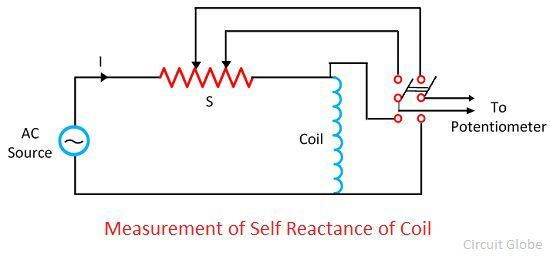# AC Potentiometer

Definition: The potentiometer which is used for measuring the phase and the magnitude of the unknown emf by comparing it with the known emf such type of potentiometer is known as the AC potentiometer. The working principle of the AC potentiometer is same as that of the DC potentiometer, i.e., the unknown voltage is determined by comparing it with the known voltage. And when both of them are equal the galvanometer indicates the null point. Hence the value of the unknown emf is known.

The operation of the AC potentiometer is quite complicated as compared to the DC potentiometer. The following are the important factor which must be considered for their operation.

1. In AC potentiometer, the frequency and the waveform of the current must be same as that of the voltage being measured. Thus, in AC potentiometer the potential is transferred from the same source as the current or voltage is measured.
2. The non-inductive element is used for making the sliding wire and the resistance coil of the AC potentiometer which reduces the reading error.
3. The reading of the AC potentiometer is affected by the external magnetic field. So, they are eliminated at the time of measurement.
4. The supply source should be sinusoidal and free from harmonics because in the presence of the harmonics the balanced is not easily achieved.

## Types of AC Potentiometer

The AC potentiometers are classified by the value measured by the potentiometer dial and scales. The AC potentiometer may be broadly classified as.

### Polar Type Potentiometer

In this potentiometer, the magnitude of the unknown voltage is measured from one scale, and its phase angle is directly on the second scale. The arrangement is made to read phase angles up to 360°. The voltage is read in the form of V∠θ.### Coordinate Type Potentiometer

The coordinate type potentiometer has two scales to read the inphase V1 component, and the quadrature component V2 of the unknown voltage V. These voltages are 90° out of phase with each other. The potentiometer is constructed in such a way so that the potentiometer read both the positive and negative value of V1 and V2 and covered all the angle up to 360°.

### Applications of Potentiometer

The AC potentiometer has numerous applications. The few of them are explained below in details.

1. Voltmeter Calibration – The AC potentiometer directly measures the low voltages up to 1.5V. The higher voltage is measured by either using the volt box ratio or two capacitors in series with the potentiometer.

2. Ammeter Calibration – The measurement of the alternating current may be measured by the use of non-inductive standard resistor with the potentiometer.

3. Wattmeter and Energy Meter Testing – The testing circuit of the Wattmeter and the energy meter is same as that of the DC measurements. The phase shifting transformer is connected to the potentiometer to vary the phase of the voltage on the current. Thus, the voltage and current may vary at different power factor.

4. Measurements of Self Reactant of a Coil – The standard reactance is placed in series with the coil whose reactance is to be measured.5. Other Applications – The AC potentiometer is used for engineering measurement in which the accuracy of 0.5 to 1% is essentials. The AC potentiometer is also used for measuring the voltage which must be resolved into two components. It gives the accurate results in magnetic testing and precise testing of instrument transformers.